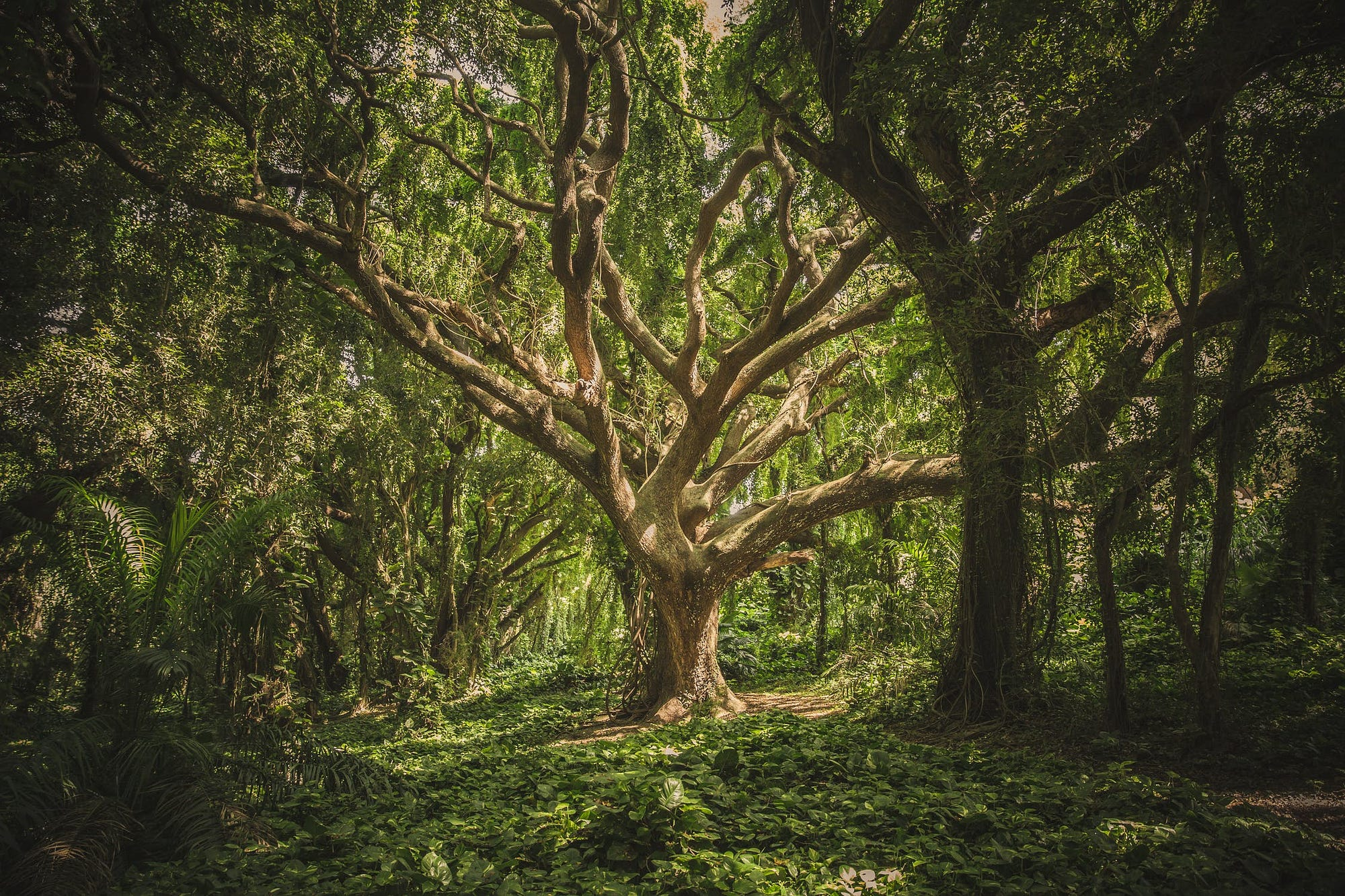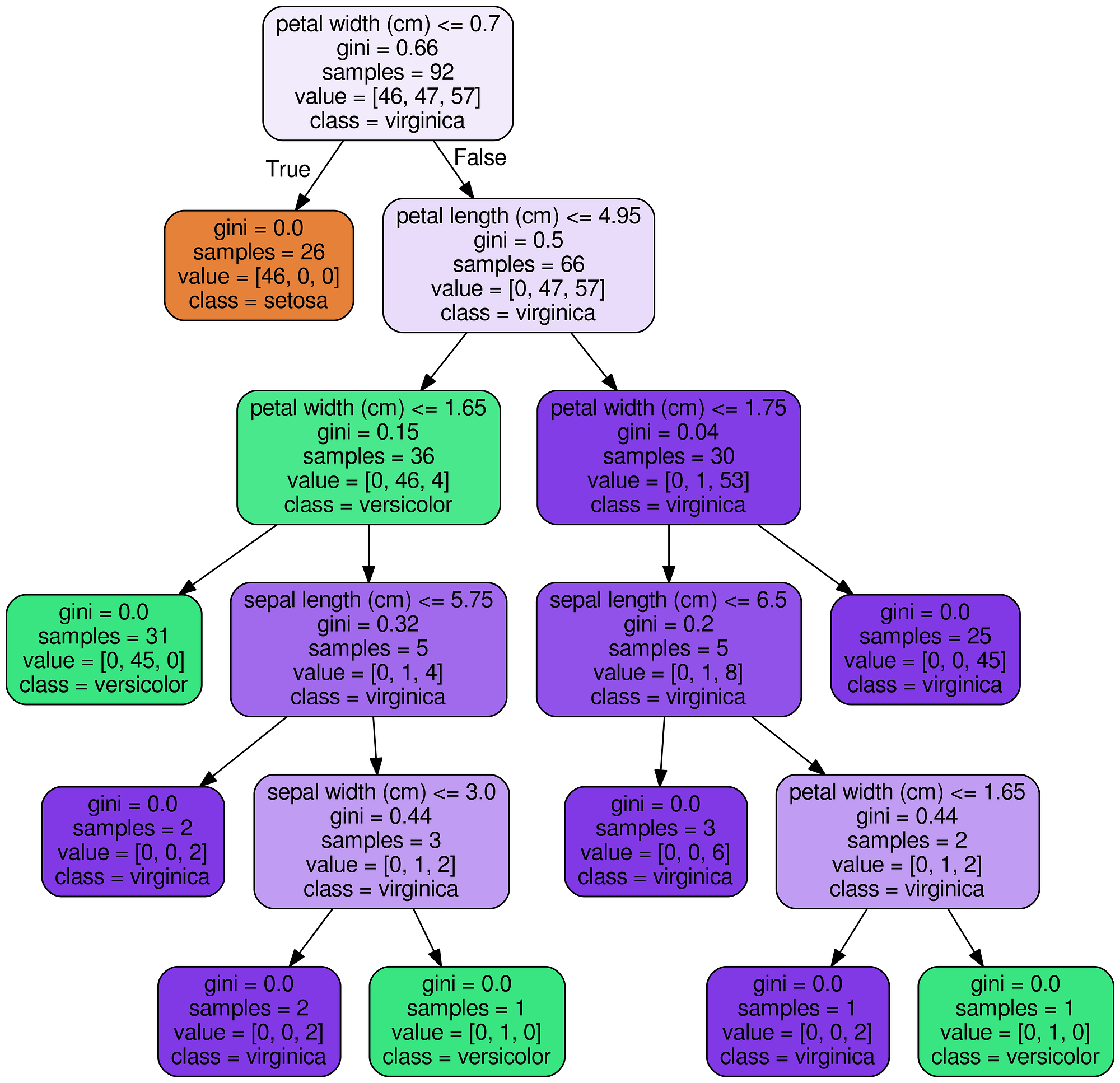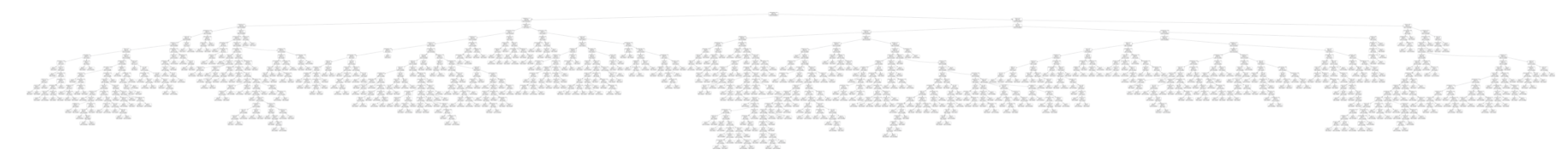# How to Visualize a Decision Tree from a Random Forest in Python Using Scikit-Learn## A helpful utility for understanding your model

Here’s the complete code: just copy and paste into a Jupyter Notebook or Python script, replace with your data and run:

Code to visualize a decision tree and save as png ([on GitHub here](https://gist.github.com/WillKoehrsen/ff77f5f308362819805a3defd9495ffd)).

The final result is a complete decision tree as an image.Decision Tree for Iris Dataset

### Explanation of code

1. Create a model train and extract: we could use a single decision tree, but since I often employ the random forest for modeling it’s used in this example. (The trees will be slightly different from one another!).

``````from sklearn.ensemble import RandomForestClassifier
model = RandomForestClassifier(n_estimators=10)
# Train
model.fit(iris.data, iris.target)
# Extract single tree
estimator = model.estimators_
``````
2. Export Tree as .dot File: This makes use of the `export_graphviz` function in Scikit-Learn. There are many parameters here that control the look and information displayed. Take a look at the documentation for specifics.

`````` from sklearn.tree import export_graphviz
# Export as dot file
export_graphviz(estimator_limited,
out_file='tree.dot',
feature_names = iris.feature_names,
class_names = iris.target_names,
rounded = True, proportion = False,
precision = 2, filled = True)
``````
3. Convert `**dot**` to `**png**` using a system command: running system commands in Python can be handy for carrying out simple tasks. This requires installation of graphviz which includes the dot utility. For the complete options for conversion, take a look at the documentation.

`````` # Convert to png
from subprocess import call
call(['dot', '-Tpng', 'tree.dot', '-o', 'tree.png', '-Gdpi=400'])
``````
4. Visualize: the best visualizations appear in the Jupyter Notebook. (Equivalently you can use `matplotlib` to show images).

`````` # Display in jupyter notebook
from IPython.display import Image
Image(filename = 'tree.png')
``````

#### Considerations

With a random forest, every tree will be built differently. I use these images to display the reasoning behind a decision tree (and subsequently a random forest) rather than for specific details.

It’s helpful to limit maximum depth in your trees when you have a lot of features. Otherwise, you end up with massive trees, which look impressive, but cannot be interpreted at all! Here’s a full example with 50 features.Full decision tree from a real problem (see here).

### Conclusions

Machine learning still suffers from a black box problem, and one image is not going to solve the issue! Nonetheless, looking at an individual decision tree shows us this model (and a random forest) is not an unexplainable method, but a sequence of logical questions and answers — much as we would form when making predictions. Feel free to use and adapt this code for your data.

As always, I welcome feedback, constructive criticism, and hearing about your data science projects. I can be reached on Twitter @koehrsen_will